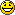# Maple TA 26_2##Maple TA 26_2

1) the # given (pos)
2) havent figure that out..
3) mu*B*cos(degrees given)=ans (pos)
4) the # given (neg)

Can someone pls post how to solve Question #2

Guest001
Guest

##number 2

for number 2

negative

e^-(given a * given x)

then square thatFor Question 3 though, i keep trying

-(magnetic moment * magnetic field * cos(given angle))

and i still am not getting the answer =/

:)
Guest

##number 3

what did you mean by m in the equation?

:)
Guest

##number 3

okies sorry for so many posts..

but i see now, you have to set your calculator to radians and after that equation, divide by (1.6x10^-19)

and ALSO my answer was negative.

cheers:)
Guest

###3

I'm still getting number three wrong. could someone explain again? thanks!

k8
Guest

##question number 3!!

IS still wrong AHHH!!

big boy
Guest

##Question number THree aid

You should not put your calculator in radians. Leave it in DEG.

Do:
(magnetic moment * magnetic field * cos(given angle))

Then divide what you get by 1.6E-19

Big Boy
Guest

##Number 4

Number 1- Obv is 2
Number 4- ABSolutely Clueless...Can anyone help me with this one? Greatly appreciate it!!!Money23
Guest

##Q #2

i used the equation posted and it didnt work for me..pls help

camp
Guest

##Re: Maple TA 26_2

Firstly, I get money. Secondly, question four requires that you haven't wasted all of your brain cells away since freshman gen chem.

Principle quantum number = n = 1,2,3,4...

Azimuthal Quant. # = l = 0,1,2,... n-1

Therefore for principle quantum number 3, l = 0,1,2 and there are therefore three predicted subshell or orbital shapes, the s, p and d shells.

CMONEY
Guest

###3 -- got it!!

so for # 3 the above formula is correct, but most of you who are not getting it is probably because you are not seeing the * 10^-24 in for the magnetic moment number.. its easy to miss since its in the left corner of the second line...
and no need to change to radians..even if you get a negative number, the answer is pos.

PHy
Guest

##Question #2

i cant seem to get Question#2..i used the equation above but it is not working ..

woo
Guest

##number 4

I for some reason cant get number 4 either. What is the answer pleaseeeee:)
Guest

##number 2

could someone please explain the logic/ breakdown of the question. I am really confused by the question itself. i get they are looking for a ration but could some one break it down for me? greatly appreciated!!!!

quantums
Guest

###3

if ur questions stated the angle in degrees set calculator to degrees, if radians then set to radians and then follow the other guys directions for #3

gamblerz
Guest

###4

and for #4 so take the negative of the number n...one significant digit is enough

gamblerz
Guest

###2

did anyone get #2

sig
Guest

##QUESTION 2

CAN SOMEONE PLS POST WHAT THEY DID FOR QUESTION NUMBER TWO..THATS ALL I NEED AND IVE BEEN WORKING ON IT FOR A WHILE NOW.

Guest001
Guest

##Question 2

this is what i understand from it (please correct me if I'm wrong!)

based on Slide 14: probability to observe particle at location x = (psi(x))^2

psi(x) = e^-(a*x)

ratio:

psi(x) = (e^-(a given * x given))^2 / (e^-(a given * 0))^2

e^-(a given * 0) = 1. Therefore, the ratio is just (e^-(a given * x given))^2

hope that helps

an0n
Guest

##Question 2 continued

ratio:

psi(x) = (e^-(a given * x given))^2 / (e^-(a given * 0))^2

ignore the crossed out part

remember, answer is negative, as posted earlier by someone else

an0n
Guest

##Re: Maple TA 26_2